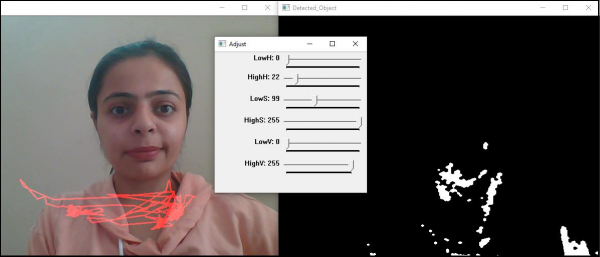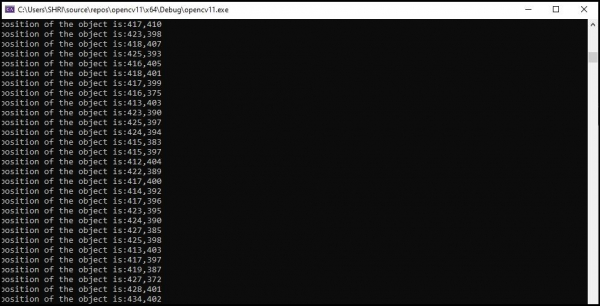# How to track the color in OpenCV using C++?

OpenCVC++Server Side ProgrammingProgramming

Color tracking is similar to color detection. For tracking purpose, we added extra few lines to calculate the area of the detected object and then track the current position of that area and finally used line() function of OpenCV to show the object's path of motion.

The following program demonstrates how to track the color in OpenCV using C++.

## Example

#include<iostream>
#include<opencv2/highgui/highgui.hpp>
#include<opencv2/imgproc/imgproc.hpp>
using namespace std;
using namespace cv;
int main(int argc, char** argv) {
VideoCapture video_load(0);//capturing video from default camera//
namedWindow("Adjust");//declaring window to show the image//
int Hue_Low= 0;//lower range of hue//
int Hue_high = 22;//upper range of hue//
int Sat_Low =99;//lower range of saturation//
int Sat_high = 255;//upper range of saturation//
int Val_Low = 0;//lower range of value//
int Val_high = 255;//upper range of value//
createTrackbar("LowH", "Adjust", &Hue_Low, 179);//track-bar for min hue//
createTrackbar("HighH","Adjust", &Hue_high, 179);//track-bar for max hue//
createTrackbar("LowS", "Adjust", &Sat_Low, 255);//track-bar for min saturation//
createTrackbar("HighS", "Adjust", &Sat_high, 255);// track-bar for max saturation//
createTrackbar("LowV", "Adjust", &Val_Low,255);//track-bar for min value//
createTrackbar("HighV", "Adjust", &Val_high, 255);// track - bar for max value//
int Horizontal_Last = -1;//initial horizontal position//
int vertical_Last = -1;//initial vertical position//
Mat temp;//declaring a matrix to load frames from video stream//
Mat track_motion = Mat::zeros(temp.size(), CV_8UC3);//creating black matrix for detection//
while (true) {
Mat actual_Image;//declaring a ,atrix for actual image//
Mat converted_to_HSV;//declaring a matrix to store converted image//
cvtColor(actual_Image, converted_to_HSV, COLOR_BGR2HSV);//converting BGR image to HSV//
Mat adjusted_frame;//declaring a matrix to detected color//
inRange(converted_to_HSV,Scalar(Hue_Low, Sat_Low, Val_Low),
Scalar(Hue_high, Sat_high, Val_high), adjusted_frame);//applying change of values of track-bars//
dilate(adjusted_frame, adjusted_frame,getStructuringElement(MORPH_ELLIPSE, Size(5, 5)));//morphological closing for filling up small holes in foreground//
erode(adjusted_frame, adjusted_frame, getStructuringElement(MORPH_ELLIPSE, Size(5, 5)));//morphological closing for filling up small holes in foreground//
Moments detecting_object = moments(adjusted_frame);//creating an object from detected color frame//
double vertical_moment = detecting_object.m01;//getting value of vertical position//
double horizontal_moment = detecting_object.m10;//getting value of horizontal position//
double tracking_area = detecting_object.m00;//getting area of the object//
if (tracking_area > 10000){ //when area of the object is greater than 10000 pixels//
int posX = horizontal_moment / tracking_area;//calculate the horizontal position of the object//
int posY = vertical_moment / tracking_area;//calculate the vertical position of the object//
if (Horizontal_Last >= 0 && vertical_Last >= 0 && posX >= 0 && posY >= 0){ //when the detected object moves//
line(track_motion, Point(posX, posY), Point(Horizontal_Last, vertical_Last), Scalar(0, 0, 255), 2);//draw lines of red color on the path of detected object;s motion//
}
Horizontal_Last = posX;//getting new horizontal position//
vertical_Last = posY;// getting new vertical position value//
}
actual_Image = actual_Image + track_motion;//drawing continuous line in original video frames//
imshow("Actual",actual_Image);//showing original video//
cout << "position of the object is:" << Horizontal_Last << "," << vertical_Last << endl;//showing tracked co-ordinated values//
if(waitKey(30)==27){ //if esc is pressed loop will break//
cout << "esc key is pressed by user" << endl;
break;
}
}
return 0;
}

## Output